International
Tables for
Crystallography
Volume B
Reciprocal space
Edited by U. Shmueli

International Tables for Crystallography (2006). Vol. B. ch. 2.4, pp. 273-274   | 1 | 2 |

## Section 2.4.4.6. Estimation of r.m.s. error

M. Vijayana* and S. Ramaseshanb

aMolecular Biophysics Unit, Indian Institute of Science, Bangalore 560 012, India, and bRaman Research Institute, Bangalore 560 080, India
Correspondence e-mail:  mv@mbu.iisc.ernet.in

#### 2.4.4.6. Estimation of r.m.s. error

| top | pdf |

Perhaps the most important parameters that control the reliability of phase evaluation using the Blow and Crick formulation are the isomorphous r.m.s. error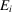and the anomalous r.m.s. error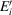. For a given derivative, the sharpness of the peak in the phase probability distribution obviously depends upon the value of E and that of E′ when anomalous-scattering data have also been used. When several derivatives are used, an overall underestimation of r.m.s. errors leads to artifically sharper peaks, the movement of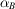towards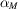, and deceptively high figures of merit. Opposite effects result when E's are overestimated. Underestimation or overestimation of the r.m.s. error in the data from a particular derivative leads to distortions in the relative contribution of that derivative to the overall phase probability distributions. It is therefore important that the r.m.s. error in each derivative is correctly estimated.

Centric reflections, when present, obviously provide the best means for evaluating E using the expression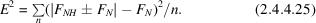As suggested by Blow & Crick (1959), values of E thus estimated can be used for acentric reflections as well. Once a set of approximate protein phase angles is available,can be calculated as the r.m.s. lack of closure corresponding to[i.e.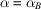in (2.4.4.20)] (Kartha, 1976).can be similarly evaluated as the r.m.s. difference between the observed anomalous difference and the anomalous difference calculated for[see (2.4.4.24)]. Normally, the value ofis about a third of that of(North, 1965).

A different method, outlined below, can also be used to evaluate E and E′ when anomalous scattering is present (Vijayan, 1981; Adams, 1968). From Fig. 2.4.2.2, we have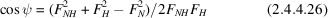and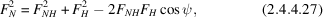where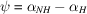. Using arguments similar to those used in deriving (2.4.3.5), we obtain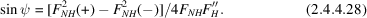If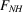is considered to be equal to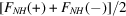, we obtain from (2.4.4.28)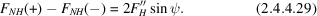We obtain what may be called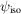if the magnitude ofis determined from (2.4.4.26) and the quadrant from (2.4.4.28). Similarly, we obtain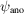if the magnitude ofis determined from (2.4.4.28) and the quadrant from (2.4.4.26). Ideally,andshould have the same value and the difference between them is a measure of the errors in the data.obtained from (2.4.4.27) usingmay be considered as its calculated value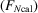. Then, assuming all errors to lie in, we may write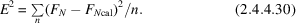Similarly, the calculated anomalous difference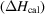may be evaluated from (2.4.4.29) using. Then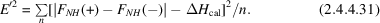If all errors are assumed to reside in, E can be evaluated in yet another way using the expression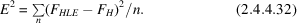### ReferencesAdams, M. J. (1968). DPhil thesis, Oxford University, England.Google ScholarBlow, D. M. & Crick, F. H. C. (1959). The treatment of errors in the isomorphous replacement method. Acta Cryst. 12, 794–802.Google ScholarKartha, G. (1976). Protein phase evaluation: multiple isomorphous series and anomalous scattering methods. In Crystallographic computing techniques, edited by F. R. Ahmed, pp. 269–281. Copenhagen: Munksgaard.Google ScholarNorth, A. C. T. (1965). The combination of isomorphous replacement and anomalous scattering data in phase determination of non-centrosymmetric reflexions. Acta Cryst. 18, 212–216.Google ScholarVijayan, M. (1981). X-ray analysis of 2Zn insulin: some crystallographic problems. In Structural studies on molecules of biological interest, edited by G. Dodson, J. P. Glusker & D. Sayre, pp. 260–273. Oxford: Clarendon Press.Google Scholar